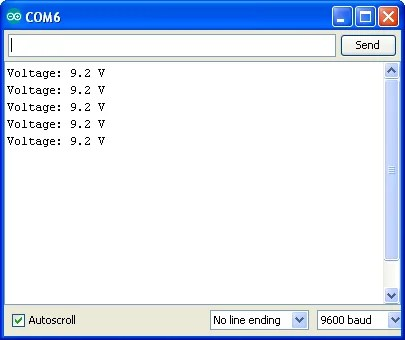# DC Voltage Measurement using Arduino

## Definition

Voltage, also called electromotive force, is a quantitative expression of the potential difference in charge between two points in an electrical field. It is measured in Volts.

## DC Voltage Measurement

Voltage measurement is the simplest task that we can perform using Arduino internal ADC.

Arduino internal ADC reference voltage is 5V (Vref=5V) so maximum voltage that we can measure without using external circuit is 5V. It is having 10-bit resolution, 210=1024 values for 0 to 5v scale. 0V corresponds to 0 ADC reading and 5V corresponds to 1023. Single ADC value represents 4.88mV i.e 1=4.88mV.

To measure higher voltages than 5V we need external voltage divider to match the ADC requirements, It converters required measurement voltage in to 0 to 5V scale. It can be created using two resistors as shown in figure 2.1. Here we are measuring 0 to 50V DC.  In this example battery is used as voltage source to be measured you can measure maximum 50V DC. In market voltage sensors are available these are just voltage divider circuits. To get the voltage reading formula is

Voltage = ADC_value x (Vref/1024) x Division Factor

Where:

Vref=5V

Division Factor is voltage divider ratio = Vin/Vout

Arduino can measure voltage with reference to ground only.

#### Components Used for DC Voltage Measurement

1. 9K, 1K Resistors
2. Arduino Uno
3. Connecting Wires

## Arduino DC Voltage Measurement Circuit

Circuit is consists of only two resistors which are used as voltage divider. Actually we measure voltage drop across the 1K Ohm resistor which is 5V when we apply 50V input across the voltage divider. As you can see that series combination of 9K and 1K is connected across the 9V battery the current flowing through the resistors is I = V / (R1+R2), in our case maximum voltage that we are measuring is 50V so maximum I = 50/10K = 5mAmp. Use of smaller values load the voltage source and affects the measurement. Use higher value resistors for better accuracy and avoid Loading Effect.

In this voltage measurement example we are measuring Voltage of the DC 9V battery, you can connect any DC source to red and black wires. This voltage measurement example can measure maximum of 50 Volts, It is useful for measurement of solar voltage, DC adapter or battery voltages. Over voltage protection circuit can be created by adding zener diode across 1K resistor. For measurement of mili Volts non inverting amplifier can be used as shown in below figure 2.3.

IC= LM358

Figure 2.3: Non Inverting Amplifier

## Arduino Code for 0 to 50V DC Voltage Measurement

```/* ReadAnalogVoltage 0 to 50 Volts - blog.circuits4you.com

result to the serial monitor.

Graphical representation is available using serial plotter   (Tools >

// the setup routine runs once when you press reset:

void setup() {

// initialize serial communication at 9600 bits per second:

Serial.begin(9600);

}

// the loop routine runs over and over again forever:

void loop() {

// read the input on analog pin 0:

// Convert the analog reading (which goes from 0 - 1023) to a voltage (0 - 50V):

float voltage = sensorValue * (5.0 / 1024.0) * 10;

// print out the value you read:

Serial.print("Voltage: ");

Serial.print(voltage);

Serial.println(" V");

delay(1000);

}```

## Results of DC Voltage Measurement

Open the Serial Monitor from (Tools>>Serial Monitor), Set Baud rate to 9600, See the voltage in serial monitor.Output on Serial Monitor